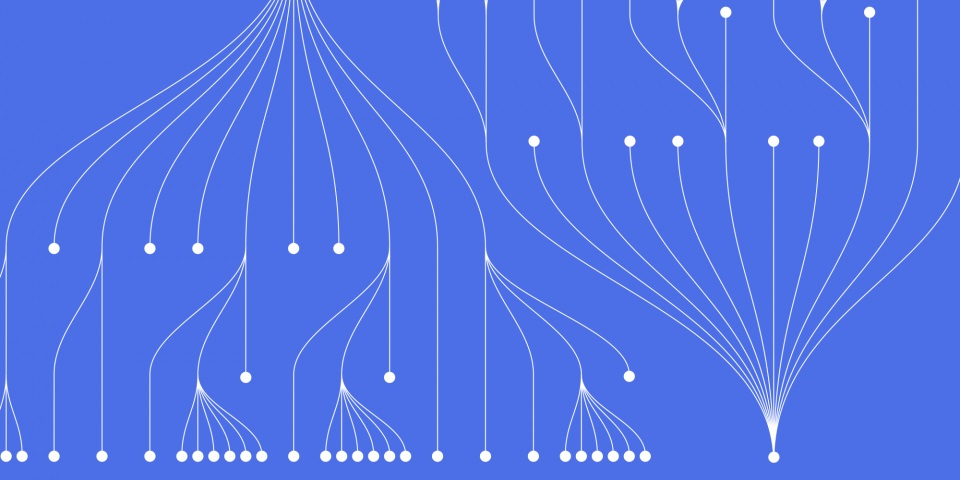18 April 2023

# How to Create a DataFrame in Scala: Step-by-Step Guide

Introduction: In Scala, a DataFrame is a distributed collection of data organized into named columns. It is one of the fundamental abstractions in Spark SQL and is used to process structured data. DataFrames can be created from various sources such as CSV, JSON, Parquet, etc. In this guide, we will learn how to create a DataFrame in Scala using various methods and sources.

Step 1: Importing Necessary Libraries Before creating a DataFrame, we need to import the necessary libraries. In Scala, we use the Spark SQL library to work with DataFrames. To import the Spark SQL library, we can use the following code:

` `

`import org.apache.spark.sql.SparkSession `

Step 2: Creating a SparkSession To create a DataFrame, we first need to create a SparkSession. A SparkSession is an entry point to any Spark functionality and provides a way to interact with Spark. To create a SparkSession, we can use the following code:

` `

`val spark = SparkSession.builder() .appName("Creating DataFrame") .master("local") .getOrCreate() `

Step 3: Creating a DataFrame from a CSV File One of the most common ways to create a DataFrame in Scala is from a CSV file. We can use the read method provided by the SparkSession object to read the CSV file and create a DataFrame. Let's assume we have a CSV file named "data.csv" with the following contents:

` `

`id,name,age 1,John,30 2,Mary,25 3,James,35 `

To create a DataFrame from this CSV file, we can use the following code:

` `

`val df = spark.read .format("csv") .option("header", "true") .option("inferSchema", "true") .load("data.csv") `

In this code, we are specifying the format of the file as CSV and setting the header and inferSchema options to true. The header option specifies that the first row of the file contains the column names, and the inferSchema option specifies that Spark should automatically infer the schema of the DataFrame from the data.

Step 4: Creating a DataFrame from a JSON File We can also create a DataFrame in Scala from a JSON file. Similar to the CSV file, we can use the read method provided by the SparkSession object to read the JSON file and create a DataFrame. Let's assume we have a JSON file named "data.json" with the following contents:

` `

`[ {"id":1,"name":"John","age":30}, {"id":2,"name":"Mary","age":25}, {"id":3,"name":"James","age":35}] `

To create a DataFrame from this JSON file, we can use the following code:

` `

`val df = spark.read .format("json") .option("inferSchema", "true") .load("data.json") `

In this code, we are specifying the format of the file as JSON and setting the inferSchema option to true to infer the schema of the DataFrame from the data.

Step 5:

To create a DataFrame from this Parquet file, we can use the following code:

` `

`val df = spark.read .format("parquet") .option("inferSchema", "true") .load("data.parquet") `

In this code, we are specifying the format of the file as Parquet and setting the inferSchema option to true to infer the schema of the DataFrame from the data.

Step 6: Creating a DataFrame from a Sequence We can also create a DataFrame in Scala from a sequence of tuples. Let's assume we have a sequence of tuples containing employee details, as follows:

` `

`val data = Seq( (1, "John", 30), (2, "Mary", 25), (3, "James", 35) ) `

To create a DataFrame from this sequence, we can use the createDataFrame method provided by the SparkSession object, as follows:

` `

`import spark.implicits._ val df = data.toDF("id", "name", "age") `

In this code, we are converting the sequence to a DataFrame by specifying the column names as "id", "name", and "age".

Step 7: Creating a DataFrame from an RDD Finally, we can create a DataFrame in Scala from an RDD (Resilient Distributed Dataset). An RDD is a fault-tolerant collection of elements that can be processed in parallel. Let's assume we have an RDD containing employee details, as follows:

` `

`val rdd = spark.sparkContext.parallelize(Seq( (1, "John", 30), (2, "Mary", 25), (3, "James", 35) )) `

To create a DataFrame from this RDD, we can use the createDataFrame method provided by the SparkSession object, as follows:

` `

`import spark.implicits._ val df = rdd.toDF("id", "name", "age") `

In this code, we are converting the RDD to a DataFrame by specifying the column names as "id", "name", and "age".

Conclusion: In this guide, we learned how to create a DataFrame in Scala using various methods and sources such as CSV, JSON, Parquet, sequences, and RDDs. We also learned how to create a SparkSession, which is an entry point to any Spark functionality. DataFrames are an essential abstraction in Spark SQL, and creating them is a fundamental skill that every Scala developer should know. By following the step-by-step instructions provided in this guide, you should now be able to create DataFrames in Scala easily.

Here are some official documentation links related to creating DataFrames in Scala:

1. Databricks Guide to Spark DataFrame - https://docs.databricks.com/getting-started/dataframes-python.html
2. Scala Documentation - https://docs.scala-lang.org/

These links provide detailed information about creating DataFrames in Scala and how to use them effectively. The Apache Spark documentation provides an in-depth explanation of the DataFrame API and examples of how to use it. The Databricks guide is a comprehensive guide to Spark DataFrames and includes best practices and tips for using them. Additionally, the Scala documentation is a great resource for learning the Scala programming language and its features.Tech Blogger & Researcher for JBI Training

CONTACT
+44 (0)20 8446 7555

[email protected]

SHAREJB International Training Ltd  -  Company Registration Number: 08458005
Registered Address: Wohl Enterprise Hub, 2B Redbourne Avenue, London, N3 2BS

POPULAR